### Very Hard GCSE Maths Problems Made Easy

Everyone knows that sinking feeling when you turn over the page and spot a mind-bendingly difficult maths problem. Encountering this in an exam is a GCSE student’s worst nightmare!

The only way to make sure that this doesn’t happen to you is to practice a variety of questions from across the syllabus and to complete as many past papers as possible. Although there’s really no shortcut, we have got a little helping hand for you in this blog post!

Our Maths guru Simon has cherry-picked a handful of the toughest problems from the most recent Edexcel GCSE Maths papers, and he’s written a step-by-step guide to solving each and every one.

Remember that in GCSE exams, question types are frequently repeated, so if you follow his methods carefully, you’ll be ready to ace these formats when they pop up in future papers!

So if you do just one thing today to benefit your Maths revision, make sure that it’s reading this post.#### Proportionality

June 2018 Paper 1H    (question 14)

y is inversely proportional to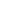.

When d =10, y =4

d is directly proportional to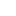When x = 2, d = 24

Find a formula for y in terms of x . Give your answer in its simplest form.

As soon as you clock that it’s a proportion question, the first thing you should aim to do is to find the both of the constants of proportionality (k)

Let’s start with the first case, y is inversely proportional to, which we can write as:

y=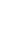(with k being the constant of proportionality).

Because we are told that y=4 when d=10, this means that we can substitute these numbers into out equation, giving us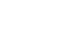This means that k is 400

Looking at the second case,(note, here the proportionality is direct rather than inverse), we can write d=k, then substitute the given values to get 24=4k

So,  k = 6 in this case.

Phew, first stage complete!

Now we have two equations,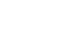and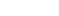But we need an equation for x in terms of y.

That means that we need to replace the d in the first equation with its value in the second equation.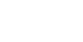Which we can simplify to: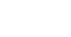and again:which is our answer.

Need some more guidance? Check out the video solutionJune 2018 Paper 1H  (question 20)

N is an integer such that 3n+2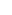14 and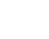> 1

Find all the possible values of n.

First things first: remember that an integer is a whole number.

Now we can start by rearranging the first inequality:

3n + 214

3n12

nNext let’s turn to the second inequality, and try to solve that to find the n values.

First multiply both sides by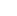+ 5

6n >+5

Now rearrange to give a quadratic

0 >– 6n + 5

0 > (n-1)(n-5)

We can then sketch the graph of this quadratic – a U-shaped parabola which cuts the x axis at 1 and 5.

1 < n < 5

We combine this with the first inequality’s rule, that n4 , to conclude that n can be 2, 3, or 4.Want to go through this again? Watch the video solution.

#### Bounds

June 2018 Paper 2H  (question 21)

Jackson is trying to find the density, in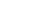, of a block of wood. The block of wood is in the same shape of a cuboid.

He measures the length as 13.2cm (correct to nearest mm), the width as 16.0cm (correct to nearest mm), and the height as 21.7cm (correct to the nearest mm).

He measures the mass as 1970g, correct to the nearest 5g.

By considering bounds, work out the density of the wood. Give your answer to a suitable degree of accuracy. You must show all your working and give a reason for your final answer.

Whoa, what a lot of information to process! Let’s break this down and attack it in parts.

We know that the overall aim is to calculate the density of the wood in.

Let’s start by calculating the upper bound (UB) and lower bound (LB) of the volume of the block.

Volume = length x width x height

Length UB = 13.15

Length LB = 13.25

Width UB= 15.95

Width LB= 16.05

Height UB= 21.65

Height LB= 21.75

Volume UB = 4625.409375

Volume LB= 4540.925125

We know the mass of the block is 1970g to the nearest 5g, meaning that the UB of the mass is 1972.5g and the LB is 1967.5g.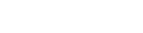so,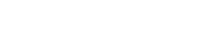Density UB= 0.43438285…

Density LB= 0.4253677…

When we write out the full answers, we see that they converge when rounded to 2 significant figures. This is why we must give our final answer as 0.43to 2 s.f. (this is a ‘suitable degree of accuracy’)

Check out the video solution to this problem here

Find our revision video on ‘Calculations using Bounds’ here

#### Trigonometry

June 2018 Paper 3H (question 19)

Here are two right-angled triangles.Given that tan e = tan f

Find the value of x. You must show all your working.

To solve this problem correctly, we must apply techniques from trigonometry and algebra.

Since we know than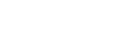we know that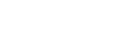and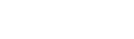We can equate the two, because we are told that tan e = tan f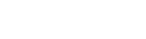Then it’s simply a matter of rearranging to solve for x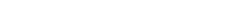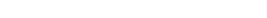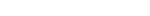Finally, we must use the quadratic formula to arrive at our final answer: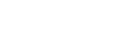This gives an x value of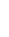(remember, lengths can’t be negative, so we ignore the negative x value)

If you’d like to see the video solution, you’ll find it hereWe hope you’ve found these worked examples helpful – keep your eyes peeled for similar questions on your exam paper, or other opportunities to put these skills and techniques into practice.

Would you like to see more blog posts like this? Let us know!

If you’re looking to continue your past paper practice session, you’ll find many more challenging questions from REAL past papers (accompanied by more step-by-step answer guides) here

If you’re looking for our Maths revision advice, you’ll find it here and here.